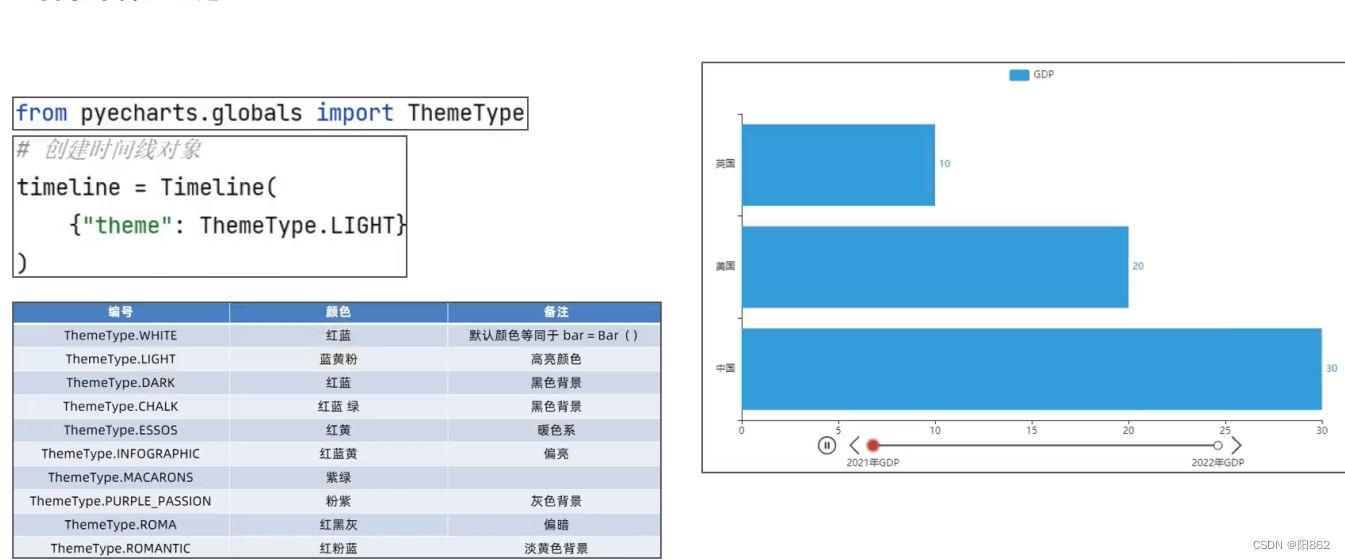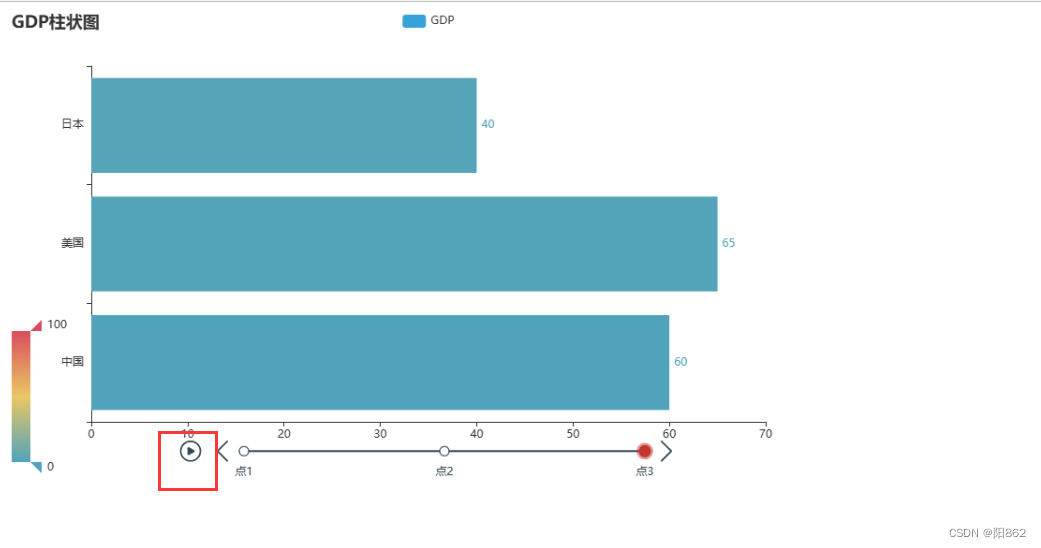﻿ Python实现动态柱状图的绘制_python_脚本之家
python# Python实现动态柱状图的绘制

## 一.基础柱状图```from pyecharts.charts import Bar
from pyecharts.options import *
#构建柱状图对象
bar=Bar()
#添加x轴对象
#添加y轴对象
#设置全局选项
bar.set_global_opts(
title_opts=TitleOpts(title="GDP柱状图"),#加名称
visualmap_opts=VisualMapOpts(#加范围显示
is_show=True
)
)
#绘图
bar.render("基础柱状图.html")``````#添加y轴对象
#反转x轴y轴
bar.reversal_axis()```• 通过Bar（）构建一个柱状图对象
• 通过柱状图对象的: reversal_axis()，反转x和y轴
• 通过label_opts=LabelOpts(position="right")设置数值标签在右侧显示

## 二.基础时间线柱状图```from pyecharts.charts import Bar,Timeline
from pyecharts.options import *
from pyecharts.globals import ThemeType
#构建柱状图对象
bar1=Bar()
#添加x轴对象
#添加y轴对象
#反转x轴y轴
bar1.reversal_axis()
#设置全局选项
bar1.set_global_opts(
title_opts=TitleOpts(title="GDP柱状图"),#加名称
visualmap_opts=VisualMapOpts(#加范围显示
is_show=True
)
)

#构建柱状图对象
bar2=Bar()
#添加x轴对象
#添加y轴对象
#反转x轴y轴
bar2.reversal_axis()
#设置全局选项
bar2.set_global_opts(
title_opts=TitleOpts(title="GDP柱状图"),#加名称
visualmap_opts=VisualMapOpts(#加范围显示
is_show=True
)
)

#构建柱状图对象
bar3=Bar()
#添加x轴对象
#添加y轴对象
#反转x轴y轴
bar3.reversal_axis()
#设置全局选项
bar3.set_global_opts(
title_opts=TitleOpts(title="GDP柱状图"),#加名称
visualmap_opts=VisualMapOpts(#加范围显示
is_show=True
)
)

#构建时间线对象
timeline=Timeline({"theme":ThemeType.LIGHT})
#在时间线上添加柱状图对象

#绘图是用时间线对象绘图，而不是用 bar了
timeline.render("基础时间线柱状图.html")``````#自动播放设置
play_interval=1000,#这里是放下一张图的时间间隔，毫秒为单位
is_timeline_show=True,#是否显示时间线
is_auto_play=True,#是否自动播放
is_loop_play=True#是否循环播放
)

#绘图是用时间线对象绘图，而不是用 bar了
timeline.render("基础时间线柱状图.html")```## 三.GDP动态柱状图绘制

1.了解列表的sort方法并配合lambda匿名函数完成列表排序

• 参数key，是要求传入一个函数，表示将列表的每一个元素都传入函数中，返回排序的依据
• 参数reverse，是否反转排序结果，True表示降序，False表示升序

```my_list=[["a",12],["b",4],["c",45]]
def choose_sort_key(element):
return element

my_list.sort(key=choose_sort_key,reverse=True)
print(my_list)

my_list.sort(key=lambda element:element,reverse=False)
print(my_list)```2.完成图表所需数据```#将数据转为字典存储，格式为：
#{ 年份:[[国家, gdp]，[国家, gdp]，...... ]，年份:[[国家,gdp]，[国家, gdp]，.....], ...... }，比如：
#{ 1960:[[美国, 123]，[中国, 231]，...... ]，1961:[[美国,124]，[中国, 234]，.....], ...... }
#先定义一个字典
data_dict={}
for line in data_lines:
year=int(line.split(","))#年份
country=line.split(",")#省份
GDP=float(line.split(","))#因为美国的GDP是科学计数法，所以用float强制转回来
#如何判断字典里面有没有制定的key呢？
try:
data_dict[year].append([country,GDP])
except KeyError:
data_dict[year]=[]
data_dict[year].append([country,GDP])

print(data_dict)```3.完成GDP动态图表绘制```from pyecharts.charts import Bar,Timeline
from pyecharts.options import *

#读取文件
f=open("D:/1960-2019全球GDP数据.csv","r",encoding="ANSI")
#关闭文件
f.close()
#删除第一行数据
data_lines.pop(0)

#将数据转为字典存储，格式为：
#{ 年份:[[国家, gdp]，[国家, gdp]，...... ]，年份:[[国家,gdp]，[国家, gdp]，.....], ...... }，比如：
#{ 1960:[[美国, 123]，[中国, 231]，...... ]，1961:[[美国,124]，[中国, 234]，.....], ...... }
#先定义一个字典
data_dict={}
for line in data_lines:
year=int(line.split(","))#年份
country=line.split(",")#省份
GDP=float(line.split(","))#因为美国的GDP是科学计数法，所以用float强制转回来
#如何判断字典里面有没有制定的key呢？
try:
data_dict[year].append([country,GDP])
except KeyError:
data_dict[year]=[]
data_dict[year].append([country,GDP])

#排序年份
sorted_year_list=sorted(data_dict.keys())
timeline = Timeline()#创建时间线对象
for year in sorted_year_list:
data_dict[year].sort(key=lambda element:element,reverse=True)
#取出本年份前八名的国家
year_data=data_dict[year][:8]
x_data=[]
y_data=[]
for country_gdp in year_data:
x_data.append(country_gdp)#x轴添加国家
y_data.append(country_gdp/100000000)#y轴添加GDP数据,单位为亿元

#构建柱状图
bar=Bar()
#反转x轴，y轴
bar.reversal_axis()

#创建时间线对象

#设置时间为自动播放
play_interval=1000,#时间间隔
is_timeline_show=True,#是否显示时间
is_loop_play=True,#是否循环
is_auto_play=True#是否自动播放
)
#绘图
timeline.render("1960——2019全球GDP前八国家.html")``````    #构建柱状图
bar=Bar()
x_data.reverse()
y_data.reverse()```from pyecharts.globals import ThemeType

timeline = Timeline({"theme":ThemeType.LIGHT})#创建时间线对象``````#反转x轴，y轴
bar.reversal_axis()
#设置每一年的图表标题
bar.set_global_opts(
title_opts=TitleOpts(title=f"{year}年全球GDP前八数据")
)```## 四.完整代码

```from pyecharts.charts import Bar,Timeline
from pyecharts.options import *
from pyecharts.globals import ThemeType

#读取文件
f=open("D:/1960-2019全球GDP数据.csv","r",encoding="ANSI")
#关闭文件
f.close()
#删除第一行数据
data_lines.pop(0)

#将数据转为字典存储，格式为：
#{ 年份:[[国家, gdp]，[国家, gdp]，...... ]，年份:[[国家,gdp]，[国家, gdp]，.....], ...... }，比如：
#{ 1960:[[美国, 123]，[中国, 231]，...... ]，1961:[[美国,124]，[中国, 234]，.....], ...... }
#先定义一个字典
data_dict={}
for line in data_lines:
year=int(line.split(","))#年份
country=line.split(",")#省份
GDP=float(line.split(","))#因为美国的GDP是科学计数法，所以用float强制转回来
#如何判断字典里面有没有制定的key呢？
try:
data_dict[year].append([country,GDP])
except KeyError:
data_dict[year]=[]
data_dict[year].append([country,GDP])

#排序年份
sorted_year_list=sorted(data_dict.keys())
timeline = Timeline({"theme":ThemeType.LIGHT})#创建时间线对象
for year in sorted_year_list:
data_dict[year].sort(key=lambda element:element,reverse=True)
#取出本年份前八名的国家
year_data=data_dict[year][:8]
x_data=[]
y_data=[]
for country_gdp in year_data:
x_data.append(country_gdp)#x轴添加国家
y_data.append(country_gdp/100000000)#y轴添加GDP数据,单位为亿元

#构建柱状图
bar=Bar()
x_data.reverse()
y_data.reverse()
#反转x轴，y轴
bar.reversal_axis()
#设置每一年的图表标题
bar.set_global_opts(
title_opts=TitleOpts(title=f"{year}年全球GDP前八数据")
)
#创建时间线对象

#设置时间为自动播放